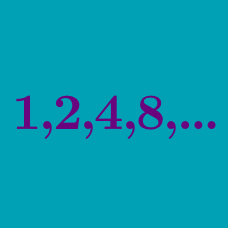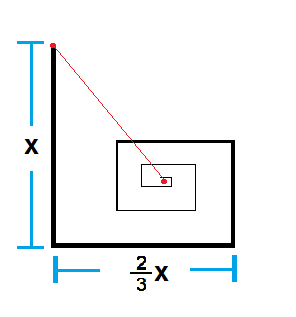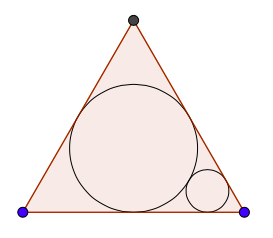Algebra

# Geometric Progressions: Level 4 ChallengesAn ant travels along a straight path with a distance of $x$ meters. From then on, it turns left and covers $\frac{2}{3}$ of the straight distance it traveled before turning, and continues doing this until it eventually reaches an unknown point $P$ (referred to as the red point in the middle of the picture). Refer to the figure above.

The distance of $P$ from the ant's starting point is $kx$ meters, where $k$ is a positive constant which can be expressed in the form $\frac{a \sqrt {b}}{c}$, where $\gcd(a,c) = 1$ and $b$ is square free. Determine the value of $a + b + c$.

Challenge: Determine an explicit formula for $k$.

If

$\large 1 + \frac{3}{x} + \frac{5}{x^2} + \frac{7}{x^3} + \frac{9}{x^4} +\ldots = 91,$

then evaluate

$\large 1 + \frac{4}{x} + \frac{9}{x^2} + \frac{16}{x^3} + \frac{25}{x^4} +\ldots$

$S= \frac{1^{2}}{10}+\frac{2 \times 1^{2}+2^{2}}{10^{2}}+\frac{3 \times 1^{2}+2 \times 2^{2}+3^{2}}{10^{3}}+\cdots$

The sum $S$ defined above is an infinite sum whose $n^\text{th}$ term is $\dfrac{n \times 1^{2}+(n-1) \times 2^{2}+(n-2) \times 3^{2} + \cdots + n^{2}}{10^{n}}$ for $n=1,2,\ldots$.

If $S$ can be expressed in the form $\frac{a}{b}$, where $a$ and $b$ are coprime positive integers, find $a+b$.You have a circle (circle 1) inscribed in an equilateral triangle. Then, you construct another circle (circle 2) that is tangent to two sides of the triangle and to circle 1. Then, you construct another circle (circle 3) that is tangent to two sides of the triangle and to circle 2. Then you construct another circle (circle 4) that is tangent to two sides of the triangle and to circle 3. Then you keep doing this infinitely. Now, not only do you do this for one angle of the triangle, but you repeat the same process for the other two angles of the triangle.

By doing the above, you get an infinite number of circles. If the radius of the circle 2 is 1, and if the area covered by all of those circles can be represented in the form $\dfrac{a \pi}{b}$, where $a$ and $b$ are coprime positive integers, find $a+b$.

If the value of

$\displaystyle \sum_{i = 0}^{\infty } \sum_{j = 0}^{\infty } \sum_{k= 0}^{\infty } \frac{1}{3^{i} 3^{j} 3^{k}}$
$(i \neq j \neq k)$

Can be represented as $\dfrac {m}{n}$

Then find

$\displaystyle m \times n$

Note: You are asked to find the summation over all ordered triplets of distinct non-negative integers.

×